#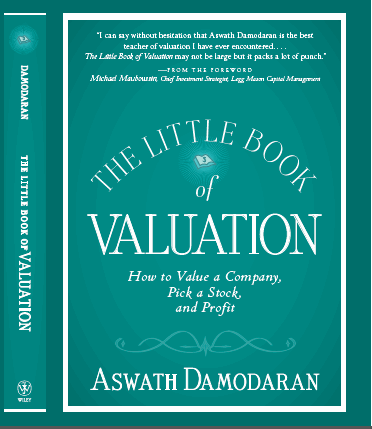The Little Book of Valuation

### Growth Rates

There is no other ingredient in discounted cash flow valuation that evokes as much angst as estimating future growth. Unlike cash flows and discount rates, where we often have the security of historical data, growth rates require us to grapple with the future. In this section, we will look first at why growth rates can be different for equity and operating earnings, examine two of the standard approaches for estimating growth (by looking at the past and using analyst estimates) and close with a discussion of the fundamentals that determine growth.

#### Equity versus Operating Earnings

As with cashflows and discount rates, a contrast has to be drawn between growth in equity earnings and growth in operating earnings. To make the distinction, consider the simplified version of an income statement in table 2.3:

Table 2.3: An Income Statement – Revenues to Earnings per Share

 Item Factors that explain differences in growth Revenues - Operating Expenses 1.     Changes in operating efficiency/ performance 2.     Operating leverage EBITDA - Depreciation & Amortization 1.     Changes in depreciation schedules/ rules 2.     Amortization of intangibles EBIT - Interest Expenses + Income from cash holdings - Taxes 1.     Changes in financial leverage (debt) 2.     Changes in cash holdings/ interest rates 3.     Changes in tax rates/ rules Net Income / Number of Shares 1.     Stock buybacks and issues 2.     Exercise of past option grants Earnings per share

We are assuming that the firm has no minority holdings in other companies, which would result in an additional line item, just above the net income line, for income from these holdings.

The growth rates in different measures of earnings (operating income, net income and earnings per share) will generally be different for most firms, and especially so for growth firms or firms in transition.

·      Share issues and Buybacks: If the number of shares remains fixed, the growth rate in earnings per share should be the same as the growth rate in net income. Firms that generate excess cash flows and use these cash flows to buy back stock will register higher growth rates in earnings per share than in net income. Conversely, firms that make a practice of raising new equity (issuing new shares) to fund investments or acquisitions can have higher growth rates in net income than in earnings per share.

·      Financial Leverage: The growth rates in operating and net income can diverge if the net interest expense (interest expense – interest income) grows at a rate different from operating income. Firms that use increasing amounts of debt to fund their operations will generally report higher growth rates in operating income than net income. However, if that debt is used to buy back shares, the earnings per share growth will reflect the fewer shares outstanding.

·      Operating Leverage: The growth in operating income can also be very different from the growth in revenues, primarily because some operating expenses are fixed and others are variable. The higher the proportion of the costs that are fixed costs (higher operating leverage), the greater will be the growth rate in operating income relative to the growth in revenues.

In effect, when asked to estimate growth rates, the first question that an analyst has to ask is “In what item?”  If our task is to estimate growth in operating income, we cannot use growth rates in earnings per share as substitutes.

#### Historical and Forecasted Growth Rates

When confronted with the task of estimating growth, it is not surprising that analysts turn to the past. In effect, they use growth in revenues or earnings in the recent past as a predictor of growth in the future. Before we put this practice under the microscope, we should add that the historical growth rates for the same company can yield different estimates n for the following reasons:

1.     Earnings measure: As we noted above, the growth rates in earnings per share, net income, operating income and revenues can be very different for the same firm over a specified time period.

2.     Period of analysis: For firms that have been in existence for long periods, the growth rates can be very different if we look at ten years of history as opposed to five years.

3.     Averaging approach: Even if we agree on an earnings measure and time period for the analysis, the growth rates we derive can be different, depending upon how we compute the values. We could, for instance, compute the growth rate in each period and average the growth rates over time, yielding an arithmetic average. Alternatively, we could use just the starting and ending values for the measure and compute a geometric average. For firms with volatile earnings, the latter can generate a very different (and lower) value for growth than the former.

A debate how best to estimate historical growth makes sense only if it is a good predictor of future growth. Unfortunately, studies that have looked at the relationship have generally concluded that (a) the relationship between past and future growth is a very weak one, (b) scaling matters, with growth dropping off significantly as companies grow and (c) firms and sectors grow through growth cycles, with high growth in one period followed by low growth in the next.

If historical growth is not a useful predictor of future growth, there is another source that we can use for future growth. We can draw on those who know the firm better than we do – equity research analysts who have tracked the firm for years or the managers in the firm – and use their estimates of growth. On the plus side, these forecasts should be based upon better information than we have available to us. After all, managers should have a clearer sense of how much they will reinvest in their own businesses and what the potential returns on investments are when they do, and equity research analysts have sector experience and informed sources that they can draw on for better information. On the minus side, neither managers nor equity research analysts are objective about the future; managers are likely to over estimate their capacity to generate growth and analysts have their own biases. In addition, both analysts and managers can get caught up in the mood of the moment, over estimating growth in buoyant times and under estimating growth in down times. As with historical growth, studies indicate that neither analyst estimates nor management forecasts are good predictors of future growth.

#### Fundamental Growth Rates

If we cannot draw on history or trust managers and analysts, how then do we estimate growth? The answer lies in the fundamentals within a firm that ultimately determine its growth rate. In this section, we will consider the two sources for growth – new investments that expand the business and improved efficiency on existing investments.

##### Decomposing Growth

The best way to consider earnings growth is to break it down algebraically into its constituent parts. Define Et to be the earnings in period t, It to be the investment at the start of period t and ROIt as the return on that investment. Thus, we can rewrite Et as:

Et = ROIt * It

The change in earnings from period t-1 to t, , DE, can then be written as follows
DE = Et – Et-1= ROIt * It - ROIt-1 * It-1

The growth rate is written in terms of DE and Et-1:

g = DE/ Et-1 = (ROIt * It - ROIt-1 * It-1)/ Et-1

Consider the simplest scenario, where the ROI is stable and does not change from period to period (ROI = ROIt = ROIt-1). The expected growth rate in earnings for this firm is:

g = DE/ Et-1 =  ROI ( It - It-1)/ Et-1

= ROI * (DI/Et-1)

In other words, the growth rate for this firm will be a function of only two variables – the return it makes on new investments (ROI) and the proportion of it’s earnings that are put into new investments (DI/Et-1).

The more general scenario is one where the return on investment does change from period to period. In this case, the expected growth rate can be written as:

g = DE/ Et-1 = ROIt * (DI/Et-1) + (ROIt – ROIt-1)/ ROIt-1

This equation is based on the assumption that the return on new investments in period t is identical to the return earned on existing investments in that period. In fact, this can be generalized even further, if we allow the return on new investments, ROINew,t, to be different from the return on existing assets, ROIExisting,t, the expected growth rate can be written as:

g = DE/ Et-1 = ROINew,t * (DI/Et-1) + (ROIExisting,t – ROIExisting,t-1)/ ROIExisting,t-1

The first term in this equation captures the growth from new investments, determined by the marginal return on those investments and the proportion invested in these investments. The second term captures the effect of changes in the return on investment on existing assets, a component that we will title “efficiency growth”. Increasing the return on investment (improving efficiency) will create additional earnings growth, whereas declining efficiency (with drops in the return on investment) will reduce earnings growth.

##### Growth from new investments

While investment and return on investment are generic terms, the way in which we define them will depend upon whether we are looking at equity earnings or operating income. When looking at equity earnings, our focus is on the investment in equity and the return is the return on equity. When looking at operating earnings, the focus is on the investment in capital and the return is the return on capital. In the cash flow definitions introduced at the start of this chapter, the change in investment is computed as the reinvestment, with the measurement of the reinvestment again varying depending upon the cash flow being discounted. In dividend discount models, reinvestment is defined as retained earnings (i.e.. any income not paid out as dividends). In free cash flow to equity (firm) models, reinvestment is defined in terms of the equity reinvestment rate (reinvestment rate).

Central to any estimate of fundamental growth is the estimate of return on capital or equity.  Table 2.4 summarizes the inputs for each measure depending on the measure of cash flow that we are focused on:

Table 2.4: Measuring Investment and Return on Investment

 Change in Investment Return on Investment Operating Income Reinvestment Rate =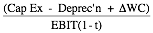Return on Invested Capital (ROC or ROIC) Net Income (Non-cash) Equity Reinvestment Rate =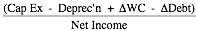Non-cash Return on Equity (NCROE) Earnings per share Retention Ratio =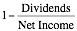Return on Equity (ROE)

It is conventional practice to use accounting measures of investment and return on investment. Thus, the book values of equity and invested capital and accounting earnings are used to compute returns on equity and capital: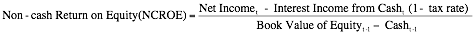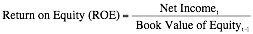The problem with accounting measures on both dimensions is well documented, with accounting choices on restructuring charges, amortization and capitalization all making a difference in the final numbers.

The final issue that we have to consider is the difference between marginal and average returns. Note that the return on investment that we use to compute the growth from new investments should be the return earned on those investments alone, i.e, a marginal return. The return on existing assets is an average return on a portfolio of investments already made. While we often use the same value for both numbers in valuation, they can be different, in fact, very different in practice.

##### Efficiency Growth

For many mature firms with limited investment opportunities, the potential for growth from new investments is limited. These firms cannot maintain a high reinvestment rate and deliver a high return on capital with that reinvestment. However, they can still grow at healthy rates if they can improve the returns that they earn on existing assets. Conversely, declines in returns on existing assets can translate into drops in earnings growth rates. Stated again in terms of different measures of earnings, efficiency growth can be written in table 2.5, as follows:

Table 2.5: Measuring Investment and Return on Investment

 Measure of return on existing assets Efficiency growth Operating Income Return on CapitalNet Income (Non-cash) Non-cash Return on EquityEarnings per share Return on Equity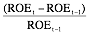When valuing companies, efficiency growth is pure gravy in terms of value created, since the growth comes with no concurrent cost. Unlike growth from new investments, where the positive effects of growth have to be offset against the negative effect of more investment, improving the return on capital on existing assets increases the growth rate without adversely affecting the cash flows. It should as come as no surprise, then, that analysts who want to increase the value of a company draw on the efficiency argument to justify much higher growth rates than those estimated using fundamentals.

While the potential for efficiency growth is always there, we should put some common sense constraints on how much we can draw on this growth.

1.     There is more potential for efficiency growth at mature firms, with poor returns on capital (equity), than there is at firms that are performing well, for two reasons. First, improving the return on capital is a much more feasible option for a firm that generates a return on capital that is well below the sector average than at a firm that already outperforms the sector.  Second, the effect of an improvement in returns on growth is much greater when the return on capital is low than when it is high. A firm that improves its return on capital from 5% to 6% will report a 20% growth rate from efficiency in that period, whereas a firm that improves its return on capital from 25% to 26% will generate a 4% growth rate from efficiency in that period.

2.     You can draw on increased efficiency to justify growth only for finite periods. After all, a firm cannot be infinitely inefficient. Once the inefficiencies, no matter how significant, are fixed, the firm will have to revert back to its sustainable growth rate, based upon new investments. In discounted cash flow valuation, this has a practical consequence: you can draw on both efficiency and new investments to justify growth during the high growth period, but only on new investments to justify growth forever (in the terminal value computation).

In closing, growth in a specific firm can come from new investments or improved efficiency, but it has to be earned either way. None of us has the power to endow companies with higher growth rates, just because we like the managers or want to make it value increase.

 To get a sense of the problems with using accounting numbers, and how best to correct for them, see: Damodaran, A., 2007, Return on capital, Return on Invested Capital and Return on Equity: Measurement and Implications, Working Paper, SSRN.